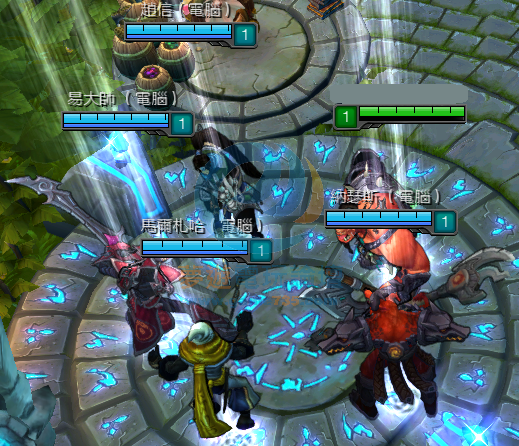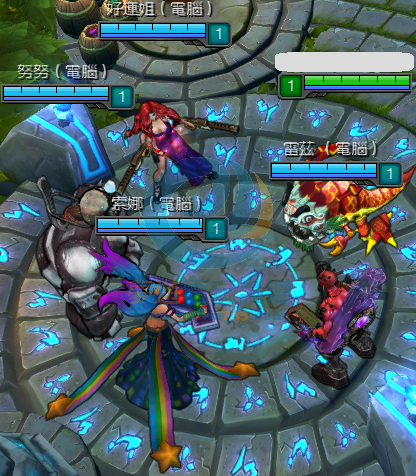•• 手機遊戲
• 線上遊戲
• PC單機遊戲
• Android 下載

### 其他# 「轉貼」英雄聯盟 免費改 英雄聯盟改SKIN小飛 ( Lv.95 九九至尊VIP ) 發表於 2014-4-3 21:26 | 顯示全部樓層 |閱讀模式Created=PlusIsLand
Site=plusisland.blogspot.tw

[Champions]
Aatrox = 1
Ahri = 4
Akali = 4
Alistar = 2
Amumu = 2
Anivia = 1
Annie = 8
Ashe = 5
Blitzcrank = 6
Brand = 4
Caitlyn = 5
Cassiopeia = 3
ChoGath = 5
Corki = 6
Darius = 3
Diana = 2
DrMundo = 6
Draven = 2
Elise = 2
Evelynn = 3
Ezreal = 5
Fiddlesticks = 6
Fiora = 3
Fizz = 3
Galio = 4
Gangplank = 1
Garen = 6
Gragas = 5
Graves = 5
Hecarim = 4
Heimerdinger = 4
Irelia = 4
Janna = 5
Jarvan IV = 5
Jax = 4
Jayce = 2
Jinx = 1
Karma = 3
Karthus = 3
Katarina = 5
Kayle = 6
Kennen = 3
KhaZix = 1
KogMaw = 5
LeBlanc = 2
LeeSin = 5
Leona = 4
Lissandra = 1
Lucian = 1
Lulu = 4
Lux = 5
Malphite = 5
Malzahar = 2
Maokai = 4
MasterYi = 3
MissFortune = 3
Mordekaiser = 4
Morgana = 5
Nami = 1
Nasus = 5
Nautilus = 3
Nidalee = 6
Nocturne = 5
Nunu = 5
Olaf = 4
Orianna = 4
Pantheon = 5
Poppy = 5
Quinn = 1
Rammus = 6
Renekton = 6
Rengar = 2
Riven = 5
Rumble = 3
Ryze = 7
Sejuani = 4
Shaco = 6
Shen = 5
Shyvana = 4
Singed = 5
Sion = 4
Sivir = 6
Skarner = 2
Sona = 5
Soraka = 3
Swain = 2
Syndra = 1
Talon = 3
Taric = 2
Teemo = 5
Thresh = 2
Tristana = 3
Trundle = 3
Tryndamere = 6
TwistedFate = 7
Twitch = 5
Udyr = 3
Urgot = 3
Varus = 2
Vayne = 4
Veigar = 7
VelKoz = 1
Vi = 1
Viktor = 3
Volibear = 3
Warwick = 6
Wukong = 3
Xerath = 3
XinZhao = 5
Yasuo = 1
Yorick = 2
Zac = 1
Zed = 1
Ziggs = 4
Zilean = 4
Zyra = 1

### 評分

zxc012343 + 1 很給力!
ccizcciz + 1 很給力!
kuo123459 + 1 很給力!
zx100500 + 1 很給力!
SAO___朔叻 + 1 低調推
kiss60230 + 2 很給力!
sd890623 + 1 很給力!
po0A0oq + 1 很給力!
s987643 + 1 低調推### 大家正在看啥SAO___朔叻 ( Lv.20 天使 ) 發表於 2014-4-7 18:37 | 顯示全部樓層
 請問如果想自己選擇 要改哪個的話 是怎麼樣弄? 從你貼的網站進去的話 那個好像只是看而已ricky0613 ( Lv.10 見習生 ) 發表於 2014-6-13 15:54 | 顯示全部樓層
 超帥!@#@!#!@@#!@#

 本版積分規則 回覆後切換到最後一頁